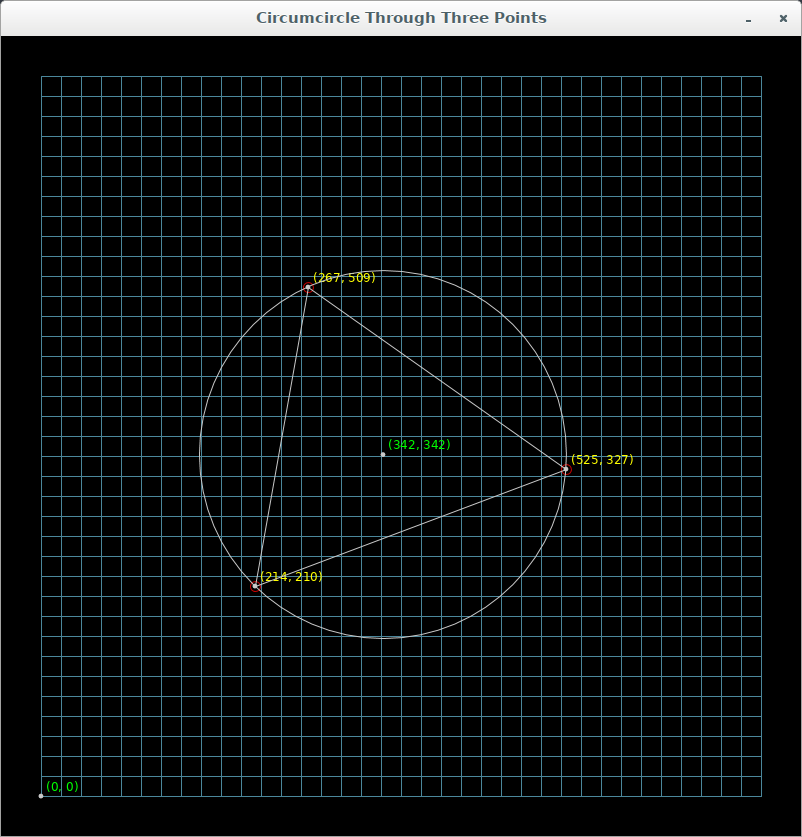For JRubyArt and propane you need to have a pretty good reason not use Vec2D and Vec3D as replacements for PVector as my recent experiments with creating a circumcircle for 3 points have confirmed. We can use a Vec2D cross product in a collinearity test (impossible with PVector). Further to determine the midpoint of two vectors is as simple as `(a + b) / 2`, you could this with PVector but it is just ugly, and furthermore you are needlessly carrying a third dimension. Here is my most recent sketch:-

### circumcircle_sketch.rb

``````
# Loosely based on a sketch by Bárbara Almeida
# https://www.openprocessing.org/sketch/179567
# Here is a sketch that clearly demonstrates some of the most egregious parts of
# vanilla processing:-
# PVector is over complicated and overloads 2D and 3D functionality, cf Vec2D
# JRubyArt and propane which behaves as a 2D vector (cross product is a float)
# and can easily be used in chain calculations.
# The inverted Y axis in processing requires remapping to use in graphs etc.
# The map method of processing can be used to do this remapping, however in many
# languages (python, ruby etc) map is a keyword with alternative usage.
# For this reason in JRubyArt and propane we have replaced vanilla processing
# map with map1d.

##################################################
# Usage click on screen with mouse to generate a point, repeat as required
# to create a triangle, and the circumcircle will be drawn unless the points are
# collinear. Additional clicks erase the first point and add a new point. To
# demonstrate collinear test press 'c' or 'C', press space-bar to clear
##################################################

attr_reader :point, :font, :points, :circle, :center

def settings
size 800, 800
# pixel_density(2) # for HiDpi screens
# smooth # see https://processing.org/reference/smooth_.html
end

def setup
sketch_title 'Circumcircle Through Three Points'
@point = MathPoint.new
@font = create_font('Arial', 16, true)
@points = TPoints.new
@renderer = AppRender.new(self)
end

def draw
graph_paper
point.display
points.map(&:display)
return unless circle
center.display
no_fill
stroke 200
render_triangle(points)
end

def mouse_pressed
points << MathPoint.new.from_sketch(mouse_x, mouse_y)
return unless points.full?
@circle = Circumcircle.new(points.array)
circle.calculate
@center = MathPoint.new(circle.center)
end

def render_triangle(points)
begin_shape
points.each do |point|
vertex(point.screen_x, point.screen_y)
end
end_shape(CLOSE)
end

def key_pressed
case key
when ' '
points.clear
when 'c', 'C'
(0..400).step(200) do |i|
points << MathPoint.new(Vec2D.new(i, i))
end
puts 'collinear points' if points.collinear?
end
end

def graph_paper
background(0)
cell_size = 20 # size of the grid
dim = cell_size * 2
(dim..width - dim).step(cell_size) do |i|
stroke(75, 135, 155)
line(i, dim, i, height - dim)
end
(dim..height - dim).step(cell_size) do |i|
line(dim, i, width - dim, i)
end
end

``````

### math_point.rb

``````
# frozen_string_literal: true
# In processing the Y-axis is inverted, plus we have a fake origin for display
# pos is the position in the Math World, which we need to translate to sketch
class MathPoint
include Processing::Proxy # to access sketch methods, including height
OR = 40.0
FORM = '(%d, %d)'.freeze
def initialize(pos = Vec2D.new)
@pos = pos
@from_mouse = false
end

def from_sketch(x, y)
@from_mouse = true
@pos = Vec2D.new(x, y))
self
end

def xpos
pos.x
end

def ypos
pos.y
end

def screen_x
pos.x
end

def screen_y
pos.y
end

def display
fill 200
no_stroke
ellipse(screen_x, screen_y, 2.5, 2.5)
display_text
return unless from_mouse
no_fill
stroke(200, 0, 0)
ellipse(screen_x, screen_y, 5, 5)
end

private

def display_text
from_mouse ? fill(255, 255, 0) : fill(0, 255, 0)
text_font(font, 12)
text(format(FORM, xpos.round, ypos.round), screen_x + 5, screen_y - 5)
end
end

``````

### points.rb

Here we create a custom enumerable class, that incorporates our collinear test, try this in java!!!

``````
# frozen_string_literal: true
require 'forwardable'
MAX_POINT = 3
# A collection of a maximum of 3 points in a Math World
# includes a collinearity test, and a map to simple Vec2D array
class TPoints
extend Forwardable
def_delegators(:@points, :each, :map, :size, :shift, :clear, :[])
include Enumerable

def initialize
@points = []
end

def <<(pt)
points << pt
shift if size > MAX_POINT
end

def collinear?
return false unless full?
(array - array).cross(array - array).zero?
end

def array
points.map(&:pos)
end

def full?
points.length == MAX_POINT
end
end

``````

### circumcircle.rb

Here we isolate the Collinear functionality in a class that has no `processing` / `java` dependencies.

``````
# frozen_string_literal: true
require 'matrix'

# Circumcircle from 3 points
class Circumcircle
def initialize(points)
@points = points
end

def calculate
@center = Vec2D.new(-(bx / am), -(by / am))
@radius = center.dist(points) # points = c
end

private

def am
2 * Matrix[
*points.map { |pt| [pt.x, pt.y, 1] }
].determinant
end

def bx
-Matrix[
*points.map { |pt| [pt.x * pt.x + pt.y * pt.y, pt.y, 1] }
].determinant
end

def by
Matrix[
*points.map { |pt| [pt.x * pt.x + pt.y * pt.y, pt.x, 1] }
].determinant
end
end

``````